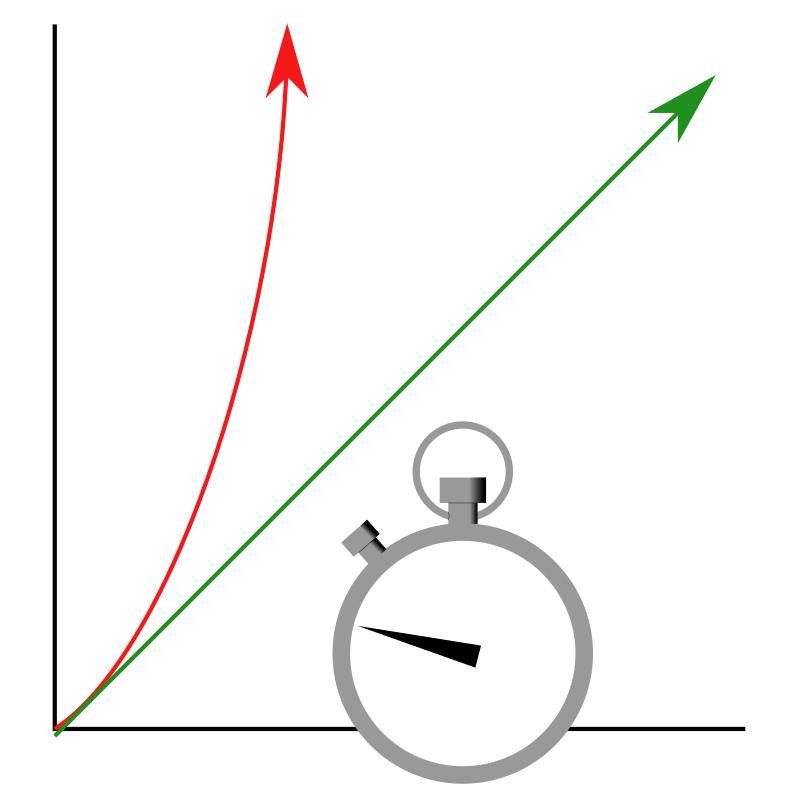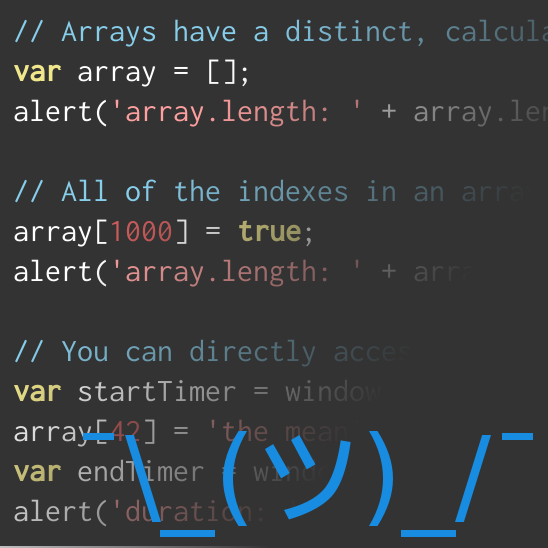## Performance Analysis is Not a Waste of TimeI recently had a conversation with a junior developer who claimed that he considered Performance Analysis to be a waste of time, so long as the code did what it was supposed to. This launched into a lesson about the costs and benefits of examining how your code will perform, and in what contexts it makes sense to spend time optimizing. The short version is that it always makes sense to think about how your code will perform; that it's a necessity any time your code becomes the bottleneck; and that it's virtually impossible to identify a bottle-neck without analyzing the code.

If you want high-performance code (if you're still reading, then you probably do) then you'll need to be able to know what poorly optimized code looks like. Sometimes, this will require you to minimize behaviors that have intrinsically poor performance, such as I/O Operations and network communication. Usually, this won't be so simple. My favorite example when explaining code with poor performance is the Fibonacci Sequence Generator. It's simple to explain, simple to write, and simple to optimize.

### The Fibonacci Sequence Generator

The Fibonacci Sequence is a mathematical pattern that most programmers and computer science majors have at least a cursory knowledge of. It is a series of numbers which starts with "0, 1" or "1, 1", where each following number is the sum of the two numbers preceding it.

/**
* Retrieves the n'th element from the Fibonacci Sequence.
*
* 0, 1, 1, 2, 3, 5, 8, 13, ...
*
* @param  {integer} n
* @return {integer}
*/
function fib(n)
{
// 0, 1
if (n <= 0) return 0;
if (n == 1) return 1;
return fib(n - 1) + fib(n - 2); // (0 + 1), (1 + 1), (1 + 2), ...
}

Simple, right? This kind of mathematical simplicity is especially alluring for functional programmers. It also conceals the amount of work that must be done to actually solve the problem. This function actually performs on the order of $O(n^2)$ — if you haven't already read my primer on asymptotic notation, that means this function performs really, really badly. The reason is that, given fib(n), the function must be called approximately $2^n$ times.### Optimization through Memoization

Memoization is an optimization technique that mixes the benefits of Lazy Loading and Caching.

/**
* Memoized fib function returns the n'th element from
* the Fibonacci Sequence more efficiently than the
* non-memoized version from earlier.
*
* 0, 1, 1, 2, 3, 5, 8, 13, ...
*
* @param  {integer} n
* @return {integer}
*/
function fib(n)
{
// 0, 1
if (n <= 0) return 0;
if (n == 1) return 1;
if (!fib.__cache[n]) {
// (0 + 1), (1 + 1), (1 + 2), ...
fib.__cache[n] = fib(n - 1) + fib (n - 2);
}
return fib.__cache[n];
}
fib.__cache = {};

By preempting the function with a check in the first line, fib(n) only ever needs to calculate a value once. Once a value has been calculated, the function will always short-circuit the return value from the cache object. This helps to preserve the readability of the function, but it also reduces the number of function calls. Since fib(5) recurses through fib(4), fib(3), and fib(2) without repeating a function call, this makes fib(n) operate at $O(n)$ and $\Omega(1)$ (if you still haven't read my primer on asymptotic notation, that's good performance).### Higher Optimization through Iteration

Some developers would consider this to be over-optimization, but fine-tuned tweaking can occasionally be useful. Some languages don't easily support the memoization technique and some languages don't handle recursion well. PHP 5.6 (for example) has especially poor performance when calling functions. If the cost of recursion becomes a bottle-neck, we can sacrifice some readability for performance by using a loop instead of recursion.

/**
* Iterated fib function returns the n'th element from
* the Fibonacci Sequence more efficiently than the
* memoized version from earlier.
*
* 0, 1, 1, 2, 3, 5, 8, 13, ...
*
* @param  {integer} n
* @return {integer}
*/
function fib(n)
{
var i;

// 0, 1
if (n <= 0) return 0;
if (n == 1) return 1;

// only calculate values that haven't already
// been calculated
for (i = fib.__cache.length; i < 1 + n; ++i) {
// (0 + 1), (1 + 1), (1 + 2), ...
fib.__cache[i] = fib.__cache[i - 2] + fib.__cache[i - 1];
}
return fib.__cache[n];
}
fib.__cache = [0, 1];

### The Reward

These optimizations can be proven with testing, and they aren't insignificant. The Memoized and Iterated versions of this function both have performance that is asymptotically equivalent to $O(n)$, but there is still a significant performance difference between all three versions. According to this test on JSPerf, a first-lookup in the Memoized Recursion vastly outstrips the unoptimized version as the value of $n$ grows. Beyond a value of approximately 25, the unoptimized version performs too slowly to be tested by my browser.

According to the same set of tests, the iterated version consistently performs twice as fast as the memoized version. The jump in performance from the recursive variant to the iterated variant is significantly less impressive than the jump from the unoptimized variant to the memoized variant.

There's really no reason to not use the memoized fib function. The benefits are drastic and obvious, it requires very little investment, and it retains much of the readability of the unoptimized version. In fact, given the profile, not using some kind of optimization feels kind of dirty.

### Summary

Unfortunately, not every problem has such a simple trade-off. I'd venture to say that most important problems are a little more complicated than this, but the Fibonacci Generator is useful for demonstrating the problems in discounting performance analysis.

Problems can be obscured by simplicity, and programmers need to be able to find a balance between elegance and performance. As a programmer, if you aren't constantly aware of the performance profile of the code you are writing, you are doing yourself (and your client) a great disservice — especially if your code is central to the system, and wrestles its overall performance to the ground based on the mistaken belief that functionality is the only thing that matters.

### Related Blog PostsTaking a look at Web AssemblyThe Tick SortCan Node use threaded Rust libraries?